Reach Us+44-1904-929220
Analysis of Second Degree of Freedom with Second Degree of Stiffness Based on Broydenand#8217;s Method | OMICS International
Journal of Civil & Environmental Engineering
All submissions of the EM system will be redirected to Online Manuscript Submission System. Authors are requested to submit articles directly to Online Manuscript Submission System of respective journal.

# Analysis of Second Degree of Freedom with Second Degree of Stiffness Based on Broyden’s Method

Department of Civil Engineering, Erbil Technical Engineering College, Erbil Polytechnic University, Erbil, Iraq

*Corresponding Author:
Department of Civil Engineering
Erbil Technical Engineering College
Erbil Polytechnic University, Erbil, Iraq
Tel: 9647504823149
E-mail: [email protected]

Received date: January 27, 2017; Accepted date: February 27, 2017; Published date: February 28, 2017

Citation: Essa S (2017) Analysis of Second Degree of Freedom with Second Degree of Stiffness Based on Broydenâ€™s Method. J Civil Environ Eng 7:266. doi:10.4172/2165-784X.1000266

Copyright: © 2017 Essa S, et al. This is an open-access article distributed under the terms of the Creative Commons Attribution License, which permits unrestricted use, distribution, and reproduction in any medium, provided the original author and source are credited.

Visit for more related articles at Journal of Civil & Environmental Engineering

#### Abstract

The main purpose of this study is comparing two methods to get the convergence of two series nonlinear spring system. The key method is Broydenâ€™s method which based on the Jacobian matrix to update the inverse of the secant stiffness matrix and the results are compared with the Newtonâ€“Raphsonâ€™s method. A constant force is applied to the second node in the system of two degrees of freedom (DOF). The results are compared with the first and second degree of nonlinear springs displacements differences that serially connected. The displacements at the first node are smaller than the second node and the number of iterations to reach the convergence for the second degree of difference displacements are greater than for the first degree. The main disadvantage of Broydenâ€™s method is that it becomes unstable when the number of iterations increases.

#### Keywords

Broyden’s method; Newton–Raphson’s method; Convergence; Iterations

#### Introduction

In general, the stiffness matrix for solid mechanics is symmetric and positive definite. However, in sometimes the updated secant matrix its inverse is not symmetric. In order to make the updated matrix symmetric and positive definite, additional constraints are required. The Broyden’s method satisfies these properties and is effectively widely used for unconstrained optimization and very suitable for finite element analysis.

The algebraic equations based on nonlinear was solved using an iterative method by Saffari and Mansouri . The nonlinear equations being linearized by evaluating the non-linear terms with the known solution from the preceding iteration. Papadrakakis  studied the application of two vector iteration methods for the large deflection behavior of spatial structures. An updated Lagrangian large rotation formulation for the governing equations based on the large deflection of nonlinear equilibrium behavior of space frames has been presented by Meek and Tan . They algorithm results are vigorous and able to handle problems that exhibit several negative eigenvalues simultaneously. A new smoothing Broyden-like method for solving the nonlinear complementarity problem was proposed by Fan . The method was based on a smoothing equation which considers the smoothing parameter as an independent variable to obtain the global convergence. Ma et al.  proposed a smoothing convergent Broyden-like method for solving nonlinear complementarity problem based on its equivalent the system of non-smooth equations. they showed that the iterates generated by the proposed method converge to a solution of the nonlinear complementarity problem globally. New semi-local convergence analysis was investigated by Argyros et al.  for an inverse free Broyden’s method in a Hilbert space setting. Gopalakrishna and Greiman  developed a method that evaluates the gradients of displacements and stresses for the structural parameters in a nonlinear finite element analysis. Lee and Park  presented an accurate numerical procedure for the generation of tangent stiffness matrices. They presented a procedure to reserve the free–free element self-equilibrium which is a criterion for convergence in the material nonlinear analysis. Kou et al.  showed a new modification of Newton method for solving nonlinear equations based on cubically convergent.

#### Theoretical Formulation

The idea of multivariable nonlinear equations (Broyden’s method) is based on the Jacobian matrix that only calculated at the first iteration, and it is updated at every iteration. The incremental solution for the ith iteration is: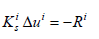(1)

where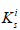is the secant stiffness matrix and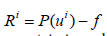residual vector at ith iteration. Then, secant stiffness matrix is updated at two successive iterations,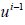and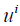. Eq (2). is the updated matrix equation.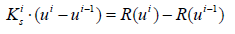(2)

Updating the stiffness matrix with minimizing its solution to the secant equation which is called Broyden’s method as: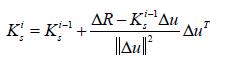(3)

where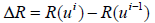and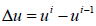. By updating the stiffness matrix, the new increment is gotten with using Eq. (1) to solve it. Later, the solution is updated based on: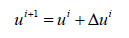(4)

The processes are repeated until the convergence criterion in Eq. (5) is satisfied.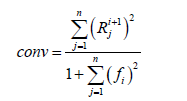(5)

Eq. (4) saves computational time throughout calculating the stiffness matrix at every iteration by updating the inverse of the secant stiffness matrix equation. Eq. (1) can be rewritten as: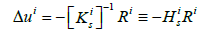(6)

where,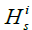is the inverse matrix that updated directly. Inverse of the secant stiffness matrix: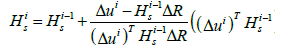(7)

Numerical example 1

For two nonlinear springs serially connected, as shown in Figure 1. The stiffness of both springs is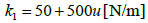and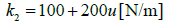with u is the elongation of the spring. The spring element equation is defined in Eq. (8) :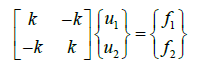(8)

Only free DOFs are used for assembly while the fixed degree of freedom (DOF) is deleted. The two stiffness of spring are assembled in matrix form in Eq. (9) :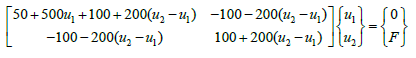(9)

u1and u2 are displacement of springs at first and second node respectively. F is applied load at the second node while the first node hasn’t any load. Eq. (10) represents a multiplying of the stiffness matrix with the unknown’s vector which leads to the system of two nonlinear equations.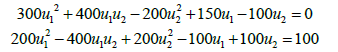(10)

By solving these equations for two DOF using Broyden’s method, the results of displacements are presented in Table 1 that shows each iteration with their convergence. The iteration halts when the convergence reaches to zero for five digits.

Iteration u1 u2 Convergence
0 0.10000 0.10000 1.01000
1 0.70000 1.70000 4.0396
2 0.30000 0.63333 0.19949
3 0.37273 0.82727 0.01766
4 0.40353 0.90941 0.00032
5 0.39989 0.89971 0.00000

Table 1: Iteration numbers with displacements and convergence for initial displacement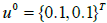for load F = 100 N using Broyden’s method.

If we used the Newton-Raphson method to compare the results, the results tabulated in Table 2 which shows the precision of this method to use it instead of others.

Iteration u1 u2 Convergence
0 0.10000 0.10000 1.01000
1 0.70000 1.70000 4.04000
2 0.46000 1.06000 0.10340
3 0.40353 0.90941 0.00032
4 0.40001 0.90004 0.00000

Table 2: Iteration numbers with displacements and convergence for initial displacementfor load F = 100 N using Newton-Raphson’s method.

Using the second degree of the stiffness with a function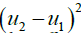instead of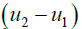in the assembled matrix equation, the stiffness matrix can be written as: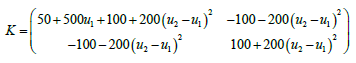(11)

The system of two nonlinear equations becomes after multiplication the stiffness matrix in Eq. (11) with unknown’s vector of displacements: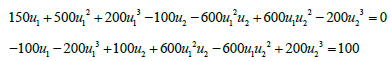(12)

Using the same procedures, the number of iteration to reach the same convergence is increased as shown in Table 3. Figures 2 and 3 show the differences between them.

Figure 2: Displacement variations with number of iterations for load 100 N for first degree of stiffness system using Broydenâ€™s method.

Figure 3: Displacement variations with number of iterations for load 100 N for second degree of stiffness system using Broydenâ€™s method.

Iteration u1 u2 Convergence
0 0.10000 0.10000 1.01000
1 0.70000 1.70000 4.03960
2 0.30000 0.63333 0.38822
3 0.34468 0.86979 0.03667
4 0.44722 1.00209 0.11766
5 0.35503 1.01547 0.23989
6 0.40518 0.97788 0.00830
7 0.39848 0.98888 0.00008
8 0.39967 0.99016 0.00002
9 0.39997 0.98977 0.00000

Table 3:Iteration numbers with displacements and convergence for initial displacementfor load F = 100 N or the second degree of stiffness using Broydenâ€™s method.

The number of iterations will decrease when the initial displacement is greater thanso that the accuracy displacements will increase as tabulated in Table 4.

Iteration u1 u2 Convergence
0 0.20000 0.20000 1.09000
1 0.48000 1.48000 6.58500
2 0.42046 0.66105 1.21700
3 0.36919 0.86826 0.07768
4 0.43827 0.99136 0.09476
5 0.37589 1.03282 0.15880
6 0.39826 0.98540 0.00006
7 0.39999 0.98948 0.00000

Table 4: Iteration numbers with displacements and convergence for initial
displacement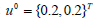for load F = 100 N for the second degree of stiffness
using Broydenâ€™s method.

Numerical example 2

To show the consistency and efficiency of this method mathematically for second degree of a function, the second polynomial of equation is taken and the results are compared between the Newton- Raphson method and Broyden’s method. The function is: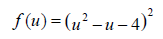(13

where, f (u) is the force varied with the displacement in one dimensional system. To determine the root of equation where the load function f (u) = 0 , the value of u = 2.56155 is satisfying the Eq. (13).

Based on Newton’s method, the function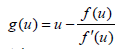and fixing the initial value of displacement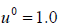. The first column in Table 5 shows the number of iterations which required to get the sufficient point for this one dimensional of force-displacement system. In addition, the third column of Table 5 represents the error up to get the actual intersection value (u = 2.56155 ). The last column of the same table is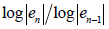which indicates the order of convergence. With implementing the Broyden’s method for the same function starting with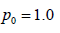and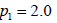by applying the equation:(14)

No. of Iteration Newton xn en Newton Convergence order
1 3.000000 0.438447 -1.850015
2 2.800000 0.238447 1.738726
3 2.686957 0.125404 1.448247
4 2.626052 0.064500 1.320236
5 2.594292 0.032739 1.247380
6 2.578050 0.016497 1.200446
7 2.569834 0.008281 1.167908
8 2.565702 0.004149 1.144178
9 2.563629 0.002077 1.126192
10 2.562592 0.001039 1.112134
11 2.562072 0.000520 1.100867
12 2.561813 0.000260 1.091646
13 2.561683 0.000130 1.083967
14 2.561618 0.000065 1.077480
15 2.561585 0.000032 1.071935
16 2.561569 0.000016 1.067156
17 2.561561 0.000008 1.063019
18 2.561557 0.000004 1.059451
19 2.561555 0.000002 1.056435

Table 5: Iteration numbers and convergence for the second degree offunction using Newton’s method with initial displacement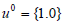with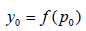and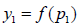. Table 6 shows the number of iterations required to achieve the same point of zero load based on the displacement results and the accuracy reaching at each step of calculation with convergence.

No. of Iteration Broydenâ€™s Method
pn
Broydenâ€™s
Method
Convergence order
1 2.000000 0.561553 -1.294760
2 2.333333 0.228220 2.560348
3 2.415385 0.146168 1.301567
4 2.476697 0.084856 1.282789
5 2.509349 0.052204 1.196935
6 2.529757 0.031796 1.167929
7 2.541986 0.019567 1.140787
8 2.549512 0.012041 1.123426
9 2.554126 0.007427 1.109336
10 2.556970 0.004583 1.098454
11 2.558723 0.002830 1.089506
12 2.559805 0.001748 1.082105
13 2.560473 0.001080 1.075840
14 2.560885 0.000667 1.070476
15 2.561140 0.000412 1.065824
16 2.561298 0.000255 1.061750
17 2.561395 0.000158 1.058152
18 2.561455 0.000097 1.054949
19 2.561493 0.000060 1.052077
20 2.561516 0.000037 1.049486
21 2.561530 0.000023 1.047132
22 2.561539 0.000014 1.044980

Table 6: Iteration numbers and convergence for the second degree of function using Broydenâ€™s method with initial displacement p0 = {1.0} .

#### Conclusion

Generally, the stiffness matrix in solid mechanics is symmetric and positive definite. However, the updated secant matrix not appears to be symmetric in Eq. (3) and its inverse in Eq. (7). In this paper, two nonlinear stiffness matrices are taken and compared. At the first, the nonlinear stiffness is first degree while in the other is second degree. Two methods are applied to get the displacements at the nodes based on Broyden’s method, the number of iterations in the first degree of stiffness system less than for that second degree to get the same convergence under the same applied load and initial displacements.

1) With increasing the number of iterations, the consistent of this method to get the final displacement is more fluctuated for the second degree of nonlinear stiffness if compared with the first degree as shown in Tables 1 and 3 with applying the Newton-Raphson’s method, the number of iteration is minimized if compared with the Broyden’s method for the same applied load and initial displacements to get the same convergence criteria that in Eq. (5). In addition, the displacements at the second node are more than for that first node in the given stiffness system.

2) With changing the initial displacements from 0.1 to 0.2, the number of iteration is reduced to get the same convergence under the identical load using Broyden’s method as shown in Table 4. If we notice the second example in section 4, the number of iterations required to achieve the same point of displacement based on the Newton-Raphson method require less number of iteration if compared with Broyden’s method.

In addition, the accuracy of the system in the second column of Table 5 is higher than for the calculated in Table 6 based on Broyden’s method. On the other hand, the order convergence of Table 5 that is based on Newton-Raphson method and the other method that is calculated in Table 6 is close to each other with the first order. Also, the key point in the Broyden’s method is that required to define the first two points at the beginning to process the function evaluations if compared with the Newton-Raphson method that is required only the first point of displacement.

#### References

Select your language of interest to view the total content in your interested language

### Article Usage

• Total views: 1186
• [From(publication date):
February-2017 - Jul 20, 2019]
• Breakdown by view type
• HTML page views : 1087Can't read the image? click here to refresh